Question

(​Magnetic Force on a Wire​) A horizontal, current-carrying wire of mass m = 40.0 g is free to slide without friction along two vertical conducting rails spaced l = 80.0 cm apart as in Figure. A uniform magnetic field of B = 1.20 T is directed into the plane of the drawing. What magnitude and direction (to the right or to the left) must the current have if the force exerted by the magnetic field on the wire is just sufficient to balance the force of gravity on the wire? (Choose to the right as the positive direction and express the magnetic field by using the sign of the result with the proper   unit. That means if you get a result of a 9.22 and the direction of the field is to the right, just type 9.22 or if you find the direction of the field as to the left just type -9.22 with the proper unit in your answer.)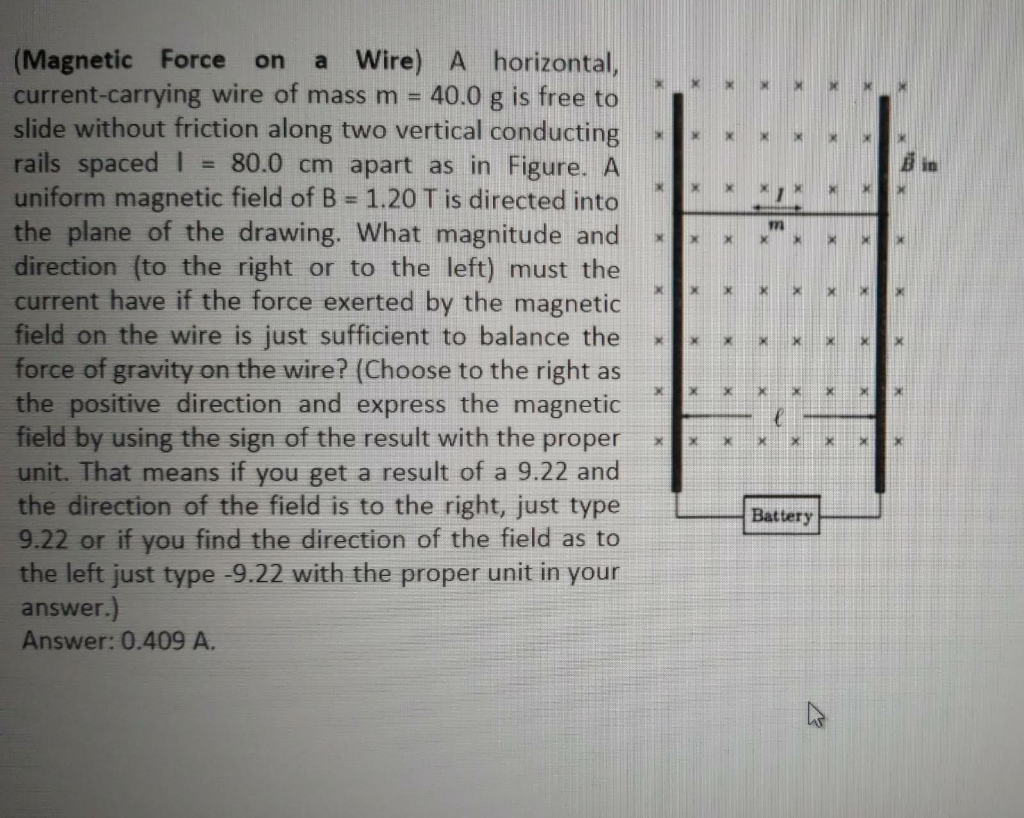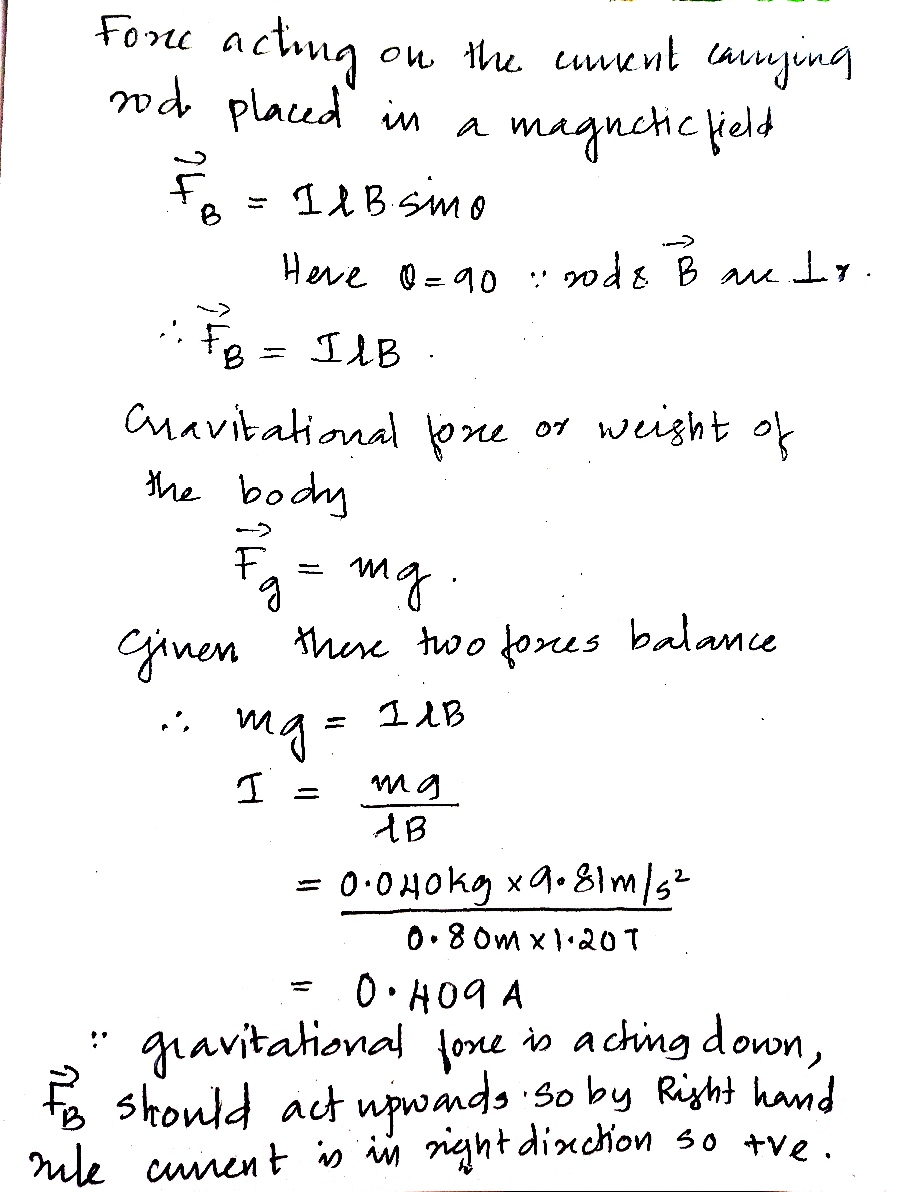#### Earn Coins

Coins can be redeemed for fabulous gifts.

Similar Homework Help Questions
• ### In what direction is the magnetic force on a current-carrying wire if the current flows to...

In what direction is the magnetic force on a current-carrying wire if the current flows to the left (-x) and the uniform magnetic field points out of the page? a. right b. left c. up d. down e. into the page f. out of the page

• ### The figure shows a stationary conducting loop adjacent to a horizontal current-carrying wire. For which of...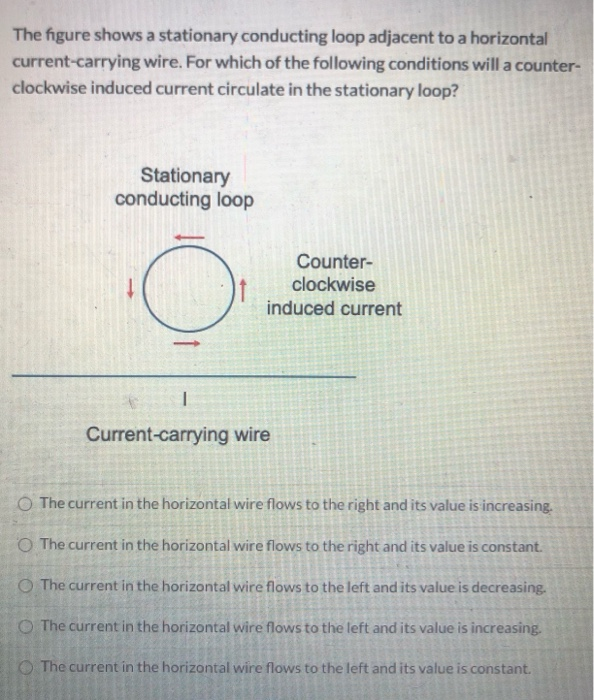The figure shows a stationary conducting loop adjacent to a horizontal current-carrying wire. For which of the following conditions will a counter- clockwise induced current circulate in the stationary loop? Stationary conducting loop O Counter- clockwise induced current - Current-carrying wire The current in the horizontal wire flows to the right and its value is increasing. The current in the horizontal wire flows to the right and its value is constant. The current in the horizontal wire flows to the...

• ### In a region with a constant magnetic field as shown, there are two conducting frictionless horizontal...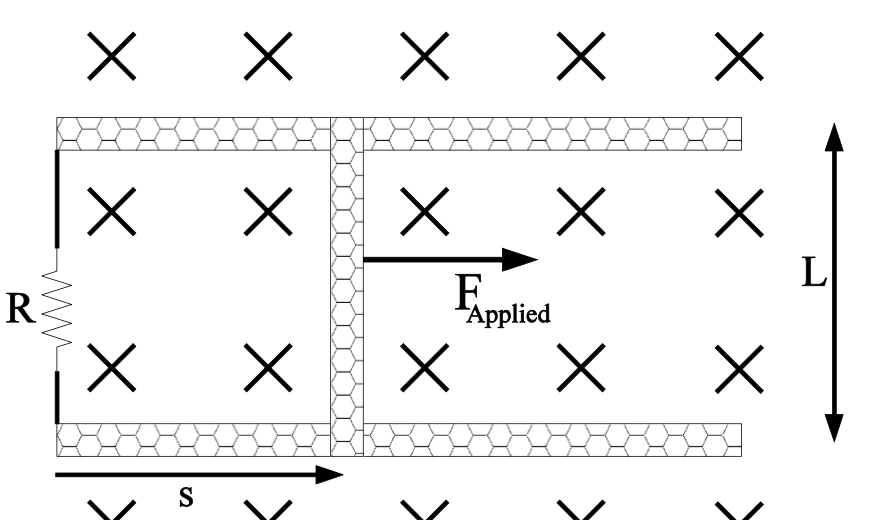In a region with a constant magnetic field as shown, there are two conducting frictionless horizontal rails, a resistor and some conducting wire along the left side and a conducting bar on the right side that is free to slide left or right. The bar is initially at rest but at time zero a force is applied to the bar pulling it to the right so that the distance s increases with time. a. Magnetic flux through the loop increases...

• ### This problem explores how a current-carrying wire can be accelerated by a magnetic field.

This problem explores how a current-carrying wire can be accelerated by a magnetic field. You will use the ideas of magnetic flux and the EMF due to change of flux through a loop. Note that there is an involved follow-up part that will be shown once you have found the answer to Part B.A.) A conducting rod is free to slide on two parallel rails with negligible friction. At the right end of the rails, a voltage source of strength...

• ### A horizontal length of current carrying wire is suspended from two identical flexible leads that are...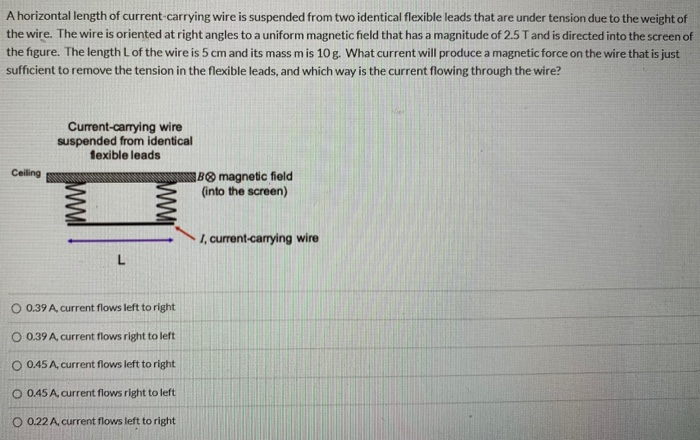A horizontal length of current carrying wire is suspended from two identical flexible leads that are under tension due to the weight of the wire. The wire is oriented at right angles to a uniform magnetic field that has a magnitude of 2.5T and is directed into the screen of the figure. The length of the wire is 5 cm and its mass mis 10g. What current will produce a magnetic force on the wire that is just sufficient to...

• ### A horizontal length of current carrying wire is suspended from two identical flexible leads that are...A horizontal length of current carrying wire is suspended from two identical flexible leads that are under tension due to the weight of the wire. The wire is oriented at right angles to a uniform magnetic field that has a magnitude of 2.5T and is directed into the screen of the figure. The length of the wire is 5 cm and its mass mis 10g. What current will produce a magnetic force on the wire that is just sufficient to...

• ### A horizontal length of current-carrying wire is suspended from two identical flexible leads that are under...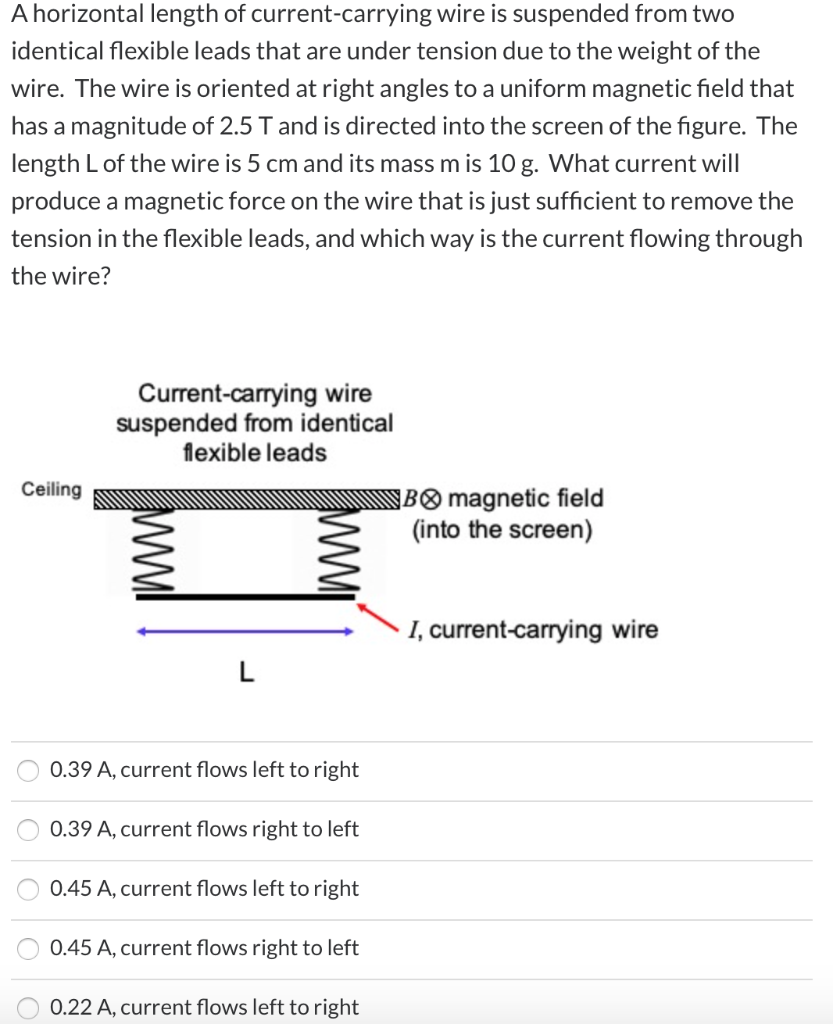A horizontal length of current-carrying wire is suspended from two identical flexible leads that are under tension due to the weight of the wire. The wire is oriented at right angles to a uniform magnetic field that has a magnitude of 2.5 T and is directed into the screen of the figure. The length L of the wire is 5 cm and its mass m is 10 g. What current will produce a magnetic force on the wire that is...

• ### A horizontal length of current-carrying wire is suspended from two identical flexible leads that are under...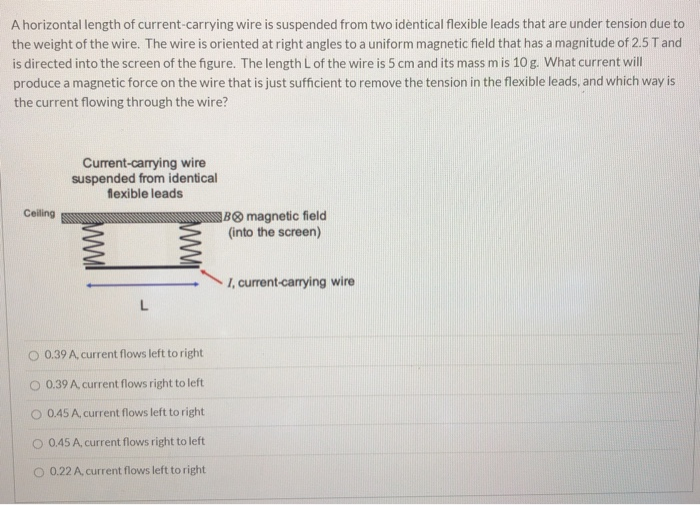A horizontal length of current-carrying wire is suspended from two identical flexible leads that are under tension due to the weight of the wire. The wire is oriented at right angles to a uniform magnetic field that has a magnitude of 2.5T and is directed into the screen of the figure. The length L of the wire is 5 cm and its mass mis 10 g. What current will produce a magnetic force on the wire that is just sufficient...

• ### A horizontal length of current-carrying wire is suspended from two identical flexible leads that are under...A horizontal length of current-carrying wire is suspended from two identical flexible leads that are under tension due to the weight of the wire. The wire is oriented at right angles to a uniform magnetic field that has a magnitude of 4T and is directed out of the screen of the figure. The length L of the wire is 5 cm and its mass mis 10 g. What current will produce a magnetic force on the wire that is just...

• ### A horizontal length of current-carrying wire is suspended from two identical flexible leads that are under...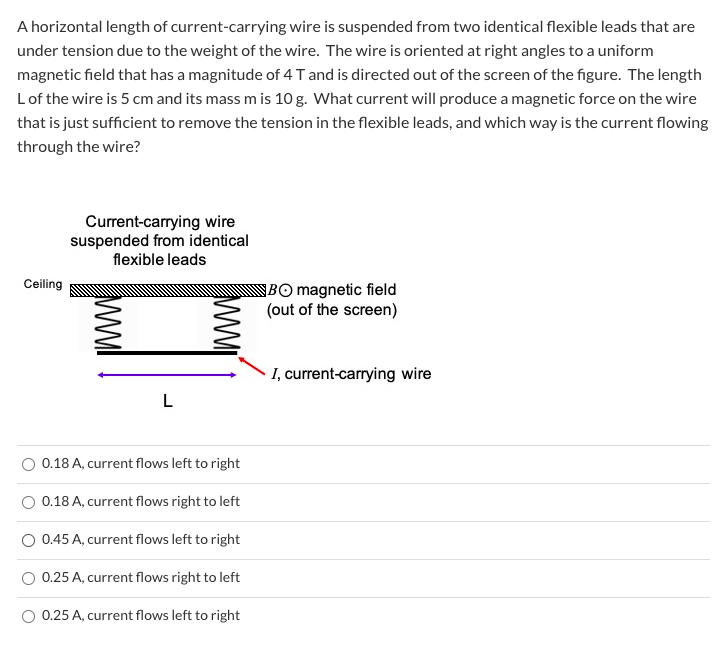A horizontal length of current-carrying wire is suspended from two identical flexible leads that are under tension due to the weight of the wire. The wire is oriented at right angles to a uniform magnetic field that has a magnitude of 4 T and is directed out of the screen of the figure. The length Lof the wire is 5 cm and its mass mis 10 g. What current will produce a magnetic force on the wire that is just...